# WKB method

(diff) ← Older revision | Latest revision (diff) | Newer revision → (diff)

An asymptotic method of G. Wentzel, H. Kramers, L. Brillouin, and H. Jeffreys for solving ordinary differential equations of the form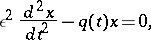(1)

with a small parameterin front of the leading derivative. The method was introduced in 1926 by these workers to obtain approximate solutions of Schrödinger's quantum-mechanical wave equation (for a detailed historical account and literature see , ). Other names given to the method are Liouville–Green approximation; the method of the phase integral; the semi-classical approximation, as well as all possible combinations of the letters W, K, B (and J).

Let,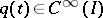andfororfor. Then there exist solutions of equation (1) such that as, uniformly in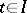,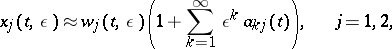and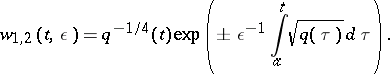(2)

The principal term of the asymptotic expansion (2) is usually called the WKB approximation.

Let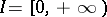, let the above conditions on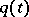be satisfied and letThen there exist solutions of equation (1) such that,, where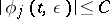for,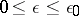, if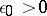is sufficiently small, and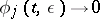if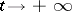,.

A pointis a turning point of equation (1) if. The WKB approximation is not valid at turning points. Asymptotic formulas valid in neighbourhoods of turning points have been obtained , . The principal term of the asymptotic expansion is expressed in the form of Bessel functions.

In a number of problems (the problem of eigenvalues, the dispersion problem) the asymptotic behaviour of a solution of equation (1) need to be known at interval ends only, i.e. asymptotics at turning points need not be found. Ifis an analytic function, it is generally possible to extend WKB formulas from one end of the intervalto the other through the complex plane(for a rigorous proof see ). For entire functionsit has been found that the WKB approximation (2) is valid in certain domains of the complex planebounded by Stokes lines (i.e. by the level linespassing through the turning points). Asymptotic formulas have been obtained for the fundamental system of solutions of equation (1) which are valid throughout the complex plane except for neighbourhoods of the turning points .

For WKB approximation of partial differential equations, see , , , , .

How to Cite This Entry:
WKB method. Encyclopedia of Mathematics. URL: http://encyclopediaofmath.org/index.php?title=WKB_method&oldid=15871
This article was adapted from an original article by M.V. Fedoryuk (originator), which appeared in Encyclopedia of Mathematics - ISBN 1402006098. See original article